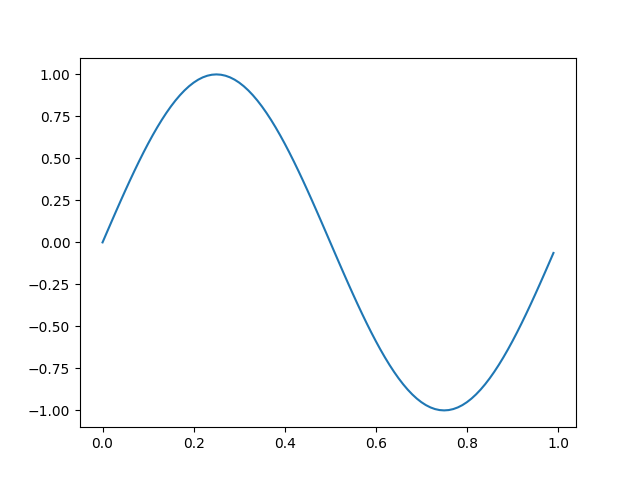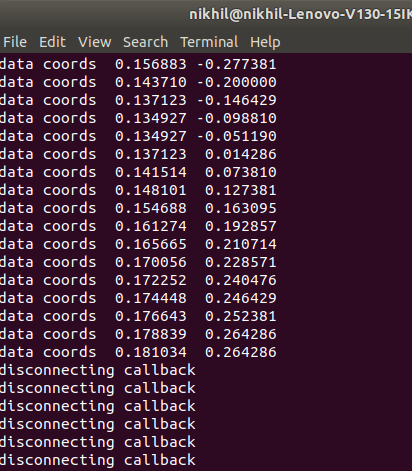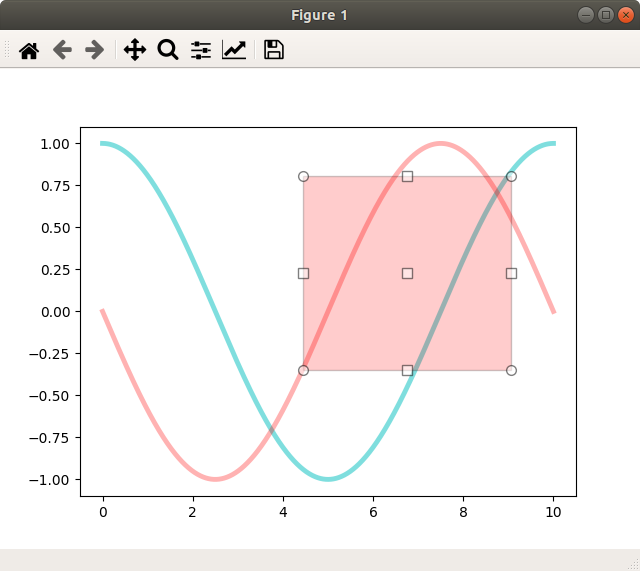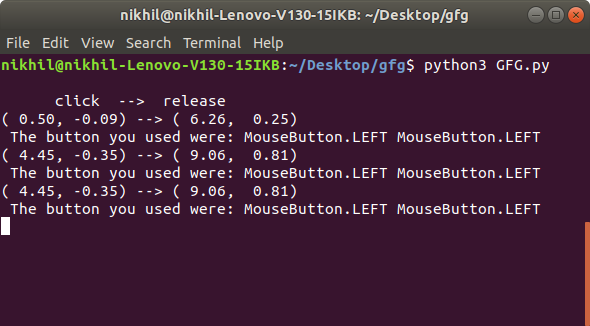# Matplotlib.pyplot.connect() in Python

Matplotlib is a library in Python and it is numerical – mathematical extension for NumPy library. Pyplot is a state-based interface to a Matplotlib module which provides a MATLAB-like interface.

## matplotlib.pyplot.connect() Function

This method is used to connect an event with string s to a function.

Syntax: matplotlib.pyplot.connect(s, func)

Parameters: This method accept the following parameters that are described below:
s(str): One of the following events ids:
1. ‘button_press_event’
2. ‘button_release_event’
3. ‘draw_event’
4. ‘key_press_event’
5. ‘key_release_event’
6. ‘motion_notify_event’
7. ‘pick_event’
8. ‘resize_event’
9. ‘scroll_event’
10. ‘figure_enter_event’,
11. ‘figure_leave_event’,
12. ‘axes_enter_event’,
13. ‘axes_leave_event’
14. ‘close_event’.
func(callable): The callback function to be executed, which must have the signature:
def func(event: Event) -> Any

Returns(cid): A connection id that can be used with FigureCanvasBase.mpl_disconnect.

Example 1 :

 `# matplotlib.pyplot.connect() ` `from` `matplotlib.backend_bases ``import` `MouseButton ` `import` `matplotlib.pyplot as plt ` `import` `numpy as np ` ` `  ` `  `t ``=` `np.arange(``0.0``, ``1.0``, ``0.01``) ` `s ``=` `np.sin(``2` `*` `np.pi ``*` `t) ` `fig, ax ``=` `plt.subplots() ` `ax.plot(t, s) ` ` `  ` `  `def` `on_move(event): ` `     `  `    ``# get the x and y pixel coords ` `    ``x, y ``=` `event.x, event.y ` `     `  `    ``if` `event.inaxes: ` `        ``ax ``=` `event.inaxes  ``# the axes instance ` `        ``print``(``'data coords % f % f'` `%` `(event.xdata, ` `                                       ``event.ydata)) ` ` `  ` `  `def` `on_click(event): ` `     `  `    ``if` `event.button ``is` `MouseButton.LEFT: ` `        ``print``(``'disconnecting callback'``) ` `        ``plt.disconnect(binding_id) ` ` `  ` `  `binding_id ``=` `plt.connect(``'motion_notify_event'``, ` `                         ``on_move) ` ` `  `plt.connect(``'button_press_event'``, on_click) ` ` `  `plt.show() `

Output :Example 2 :

 `from` `matplotlib.widgets ``import` `RectangleSelector ` `import` `numpy as np ` `import` `matplotlib.pyplot as plt ` ` `  ` `  `def` `line_select_callback(eclick, erelease): ` `     `  `    ``# Callback for line selection. ` `    ``# *eclick * and * erelease * ` `    ``# are the press and release events. ` `    ``x1, y1 ``=` `eclick.xdata, eclick.ydata ` `    ``x2, y2 ``=` `erelease.xdata, erelease.ydata ` `    ``print``(``"(% 3.2f, % 3.2f) --> (% 3.2f, % 3.2f)"` `%` `(x1, y1, x2, y2)) ` `    ``print``(``" The button you used were: % s % s"` `%` `(eclick.button,  ` `                                                  ``erelease.button)) ` ` `  ` `  `def` `toggle_selector(event): ` `     `  `    ``print``(``' Key pressed.'``) ` `     `  `    ``if` `event.key ``in` `[``'Q'``, ``'q'``] ``and` `toggle_selector.RS.active: ` `        ``print``(``' RectangleSelector deactivated.'``) ` `        ``toggle_selector.RS.set_active(``False``) ` `        ``print``(``' RectangleSelector activated.'``) ` `        ``toggle_selector.RS.set_active(``True``) ` ` `  ` `  `# make a new plotting range ` `fig, current_ax ``=` `plt.subplots() ` ` `  `# If N is large one can see ` `N ``=` `100000` ` `  `# improvement by use blitting !  ` `# plt.plot(x, +np.sin(.2 * np.pi * x),  ` `# lw = 3.5, c ='b', alpha =.7)   ` `# plot something ` `x ``=` `np.linspace(``0.0``, ``10.0``, N) ` `plt.plot(x, ``+``np.cos(.``2` `*` `np.pi ``*` `x),  ` `         ``lw ``=` `3.5``, c ``=``'c'``, alpha ``=``.``5``) ` `plt.plot(x, ``-``np.sin(.``2` `*` `np.pi ``*` `x),  ` `         ``lw ``=` `3.5``, c ``=``'r'``, alpha ``=``.``3``) ` ` `  `print``(``"\n      click  -->  release"``) ` ` `  `# drawtype is 'box' or 'line' or 'none' ` `toggle_selector.RS ``=` `RectangleSelector(current_ax, line_select_callback, ` `                                       ``drawtype ``=``'box'``, ` `                                       ``useblit ``=` `True``, ` `                                       ``button ``=``[``1``, ``3``],  ``# don't use middle button ` `                                       ``minspanx ``=` `5``, minspany ``=` `5``, ` `                                       ``spancoords ``=``'pixels'``, ` `                                       ``interactive ``=` `True``) ` ` `  `plt.connect(``'key_press_event'``, toggle_selector) ` `plt.show() `

Output :My Personal Notes arrow_drop_upCheck out this Author's contributed articles.

If you like GeeksforGeeks and would like to contribute, you can also write an article using contribute.geeksforgeeks.org or mail your article to contribute@geeksforgeeks.org. See your article appearing on the GeeksforGeeks main page and help other Geeks.

Please Improve this article if you find anything incorrect by clicking on the "Improve Article" button below.

Article Tags :

Be the First to upvote.

Please write to us at contribute@geeksforgeeks.org to report any issue with the above content.